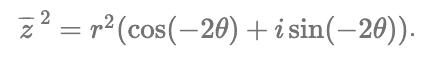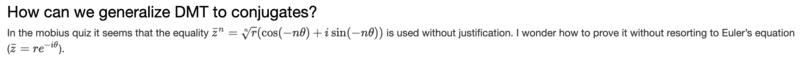# Power of a complex conjugate

• I
Leo LiuCan someone please tell me why this is true? This isn't exactly the De Moivre's theorem. Thank you.

#### Attachments

Homework Helper
Gold Member
2022 Award
If you try to justify that formula, where do you get stuck?

•Leo Liu
Leo Liu
$$\bar z^2=(x-yi)^2$$
$$\bar z^2=r^2(\cos\theta-i\sin\theta)^2$$
$$\bar z^2=r^2(\cos(2\theta)-i\sin(2\theta))$$
$$\bar z^2=r^2(\cos2\theta+i\sin(-2\theta))$$
$$\bar z^2=r^2(\cos(-2\theta)+i\sin(-2\theta))$$
I got it. Thanks.

Homework Helper
Gold Member
2022 Award
$$\bar z^2 = (re^{-i\theta})^2 = r^2e^{-2i\theta} =r^2(\cos (-2\theta) +i\sin(-2\theta))$$

••hutchphd, Leo Liu and FactChecker
Leo Liu
$$\bar z^2 = (re^{-i\theta})^2 = r^2e^{-2i\theta} =r^2(\cos (-2\theta) +i\sin(-2\theta))$$
I actually stated that I didn't want to use this method on the course chat haha.Thank you though! It is very neat.

Homework Helper
Gold Member
I actually stated that I didn't want to use this method on the course chat haha.
View attachment 293673
Thank you though! It is very neat.
You should study each part you doubt until it is intuitive to you. It is very fundamental and important.
In words explain why:
If ##z=re^{i\theta}##, why does ##\bar{z}=re^{-i\theta}##?
Then why does ##\bar{z}^2=re^{-i2\theta}##?
etc.

•PeroK
Homework Helper
Gold Member
Many mathematicians consider Euler's Formula, ##e^{i\theta} = \cos{\theta} + i\sin{\theta}##, to be the most important equation in mathematics. You should get very comfortable with using it.

Last edited:
•Leo Liu
You can also just to algebra on the conjugate expressed in terms of ##\theta##, and then apply double angle trig formulas, if you want to see it verified brute force. It works out very straightforward this way, though, of course, using exponentials is far more elegant.

Leo Liu
If z=reiθ, why does z¯=re−iθ?
I guess it's because $$\text{cis}(-\theta)=e^{-i\theta}$$.I guess it's because $$\text{cis}(-\theta)=e^{-i\theta}$$.•